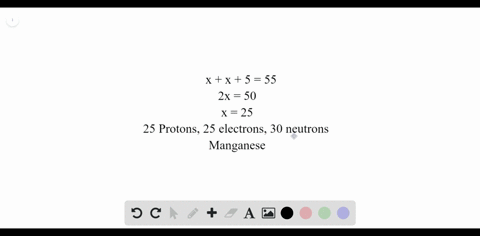### Challenge An atom has a mass number of 55. Its nu…

00:52University of Miami
Problem 16

Determine the number of protons, electrons, and neutrons for isotopes b.-f. in the table above
Name each isotope, and write its symbol.

The five elements are calcium- 46$\left(\frac{46}{20} \mathrm{Ca}\right)$ $\operatorname{axygen}-17\left(\frac{17}{8} \mathrm{O}\right),$ iron-5 7$\left( \begin{array}{c}{57} \\ {26}\end{array}\right)$ $\operatorname{zinc}-64\left(\frac{64}{30} \mathrm{Zn}\right),$ and mercury-204 $\left(\frac{204}{80} \mathrm{Hg}\right)$

## Discussion

You must be signed in to discuss.

## Video Transcript

and I will work on problem 16 from chapter four. This problem. We're asked to determine the number of protons, electrons and neutrons for isotopes listed at a table. And we need to name each isotope and write its symbol. So first, we can produce the table here. So first we have element. Uh, we have from the table the atomic number and mass number. We want to know the number of protons, number of electrons, number of neutrons, and then the name of the ascent Hope and it's symbol. So first we concert with first Element, which is calcium. We have calcium first, after that will work on oxygen. Then we have iron, zinc and mercury. So calcium, the, uh, atomic number and mass number which were given you're 20 and 46 so atomic number should equal to the number of protons and number of electrons we have. 20 of those number of neutrons will be the mass number, minus the atomic number. So it's 26 since the mass number is the number of protons and neutrons combined. So the name for this ice Ato is calcium, followed by the mass number 46 and then the symbol will be 46 20 c a. For oxygen. We have atomic number eight mass number of 17 number of protons and electrons should be eight. Number of neutrons is the number of mass number minus the atomic number, which is nine. We have oxygen 17 with mass number 17 0 and atomic number of Hey and has ah, atomic number of 26 mass number of 57 number of protons and electrons is 26. Number of neutrons is 57 minus 26 or 31. So we have iron 57 and then we can write this symbol here 57 26 e for iron zinc, atomic number of 30 mass number 64 Protons and electrons is equal to the atomic number. Number of neutrons is equal to 64 minus 30 The name of this isotope zinc 60 or and we have mass number of 64 atomic number of 30 zinc. Finally, we have mercury, which has an a top number of 80 mass number two of four for this ice. It number of protons and electrons is 80. A number of neutrons is 124. We have a name of this ice Ato is Mercury 204 and our symbol will be to a four for the mass number 80 for the atomic number and it's G or are simple.